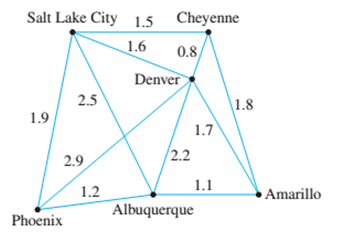Chapter 10.6, Problem 11ES### Discrete Mathematics With Applicat...

5th Edition
EPP + 1 other
ISBN: 9781337694193

#### Solutions

Chapter
Section### Discrete Mathematics With Applicat...

5th Edition
EPP + 1 other
ISBN: 9781337694193
Textbook Problem
6 views

# A pipeline is to be built that will link six cities. The cost (in hundreds of millions of dollars) of constructing each potential link depends on distance and terrain and is shown in the weighted graph below. Find a system of pipelines to connect all the cities and yet minimize the total cost.To determine

Find a system of pipelines to connect all the cities and yet minimize the total cost.

Explanation

Given info:

A pipeline is to be built that will link six cities. The cost (in hundreds of millions of dollars) of constructing each potential link depends on distance and terrain and is shown in the weighted graph below:

Calculation:

Kruskal’s algorithm:

• Start from a graph T that contains only the vertices and no edges.
• Repeatedly select the edge in the graph G with the smallest weight (that doesn’t cause a circuit) and add it to the graph T.
• Once the graph is connected. We have found a minimum spanning tree. We ignore all edges that were previously added to the graph.

First iteration: The edge with the smallest weight is the edge between Cheyenne and Denver with weight 0.8, thus we add the edge [Cheyenne, Denver] to the minimum spanning tree.

Second iteration: The edge with the smallest weight is the edge between Amarillo and Albuquerque with weight 1.1, thus we add the edge [Albuquerque, Amarillo] to the minimum spanning tree.

Third iteration: The edge with the smallest weight is the edge between Phoenix and Albuquerque with weight 1.2, thus we add the edge [Albuquerque, Phoenix] to the minimum spanning tree.

Fourth iteration: The edge with the smallest weight is the edge between Cheyenne and Salt Lake City with weight 1

### Still sussing out bartleby?

Check out a sample textbook solution.

See a sample solution

#### The Solution to Your Study Problems

Bartleby provides explanations to thousands of textbook problems written by our experts, many with advanced degrees!

Get Started